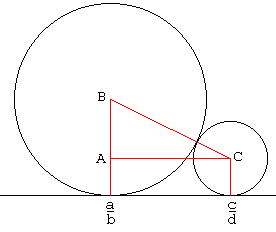# Ford's touching circles

For the Ancients who did not possess a proper symbolism for either specific (arithmetic) or generic (algebra) numbers, calculations were carried out in a geometric framework. A product of two numbers was represented by the area of a rectangle whose sides were given by the two numbers. Algebra and arithmetic were finally separated from Geometry with the systematic introduction of algebraic notations that began only in the sixteenth century. In the seventeenth century, Rene Descartes (1596-1650) invented Analytic Geometry and claimed that any geometric problem can be solved algebraically. (Which is only true in principle because reduction of a geometric problem often leads to algebraic equations that we only know to solve approximately.) Still Mathematics went its own way and nowadays Algebra and Geometry are considered completely independent sciences. But links between the two pop up now and then and many are quite unexpected in their simplicity. [Conway and Guy, p. 153] credit Lester R. Ford with the following example.Consider a straight line to serve as an x-axis in the plane. For any rational number (and a point on x-axis) p/q, draw a circle of diameter 1/q2 directly above the point p/q. Some of these circles touch, some do not. If two circles corresponding to fractions a/b and c/d touch then the circle corresponding to the mediant fraction (a+c)/(b+d) is tangent to them both.The proof is based on the fact that the two circles corresponding to a/b and c/d touch iff ad and bc are consecutive integers. The diagram above is helpful in proving the latter fact. However, a complete proof would require looking into several cases of possible relations between the four integers involved. On the right, the diagram has been drawn with the assumptions that b < d and a/b < c/d.

Form the right triangle ABC. Then, by construction, 2·AB = 1/b2 - 1/d2, 2·BC = 1/b2 + 1/d2, and AC = c/d - a/b. From the Pythagorean Theorem it follows that bc - ad = 1, as needed.

The argument is reversible. If AC and AB are defined as above then the length of the line BC (the line connecting centers of the two circles) will be equal to the sum of their radii. Which directly implies that the circles are tangent to each other.

Since bc - ad = 1 implies both (b+d)c - (a+c)d = 1 and (a+c)b - (b+d)a = 1 the mediant circle touches the other two iff they are tangent to each other. Thus we may continue adding touching circles at the points defined by successive Farey series.

## Reference

1. J. H. Conway and R. K. Guy, The Book of Numbers, Springer-Verlag, NY, 1996.### Related materialRead more...

• Chessboard
• Solitaire on a Circle
• Peg Solitaire
• Euclid's Game
• Sam Loyd's fifteen
• Swapping Rows and Columns
• Escape of the Clones
•